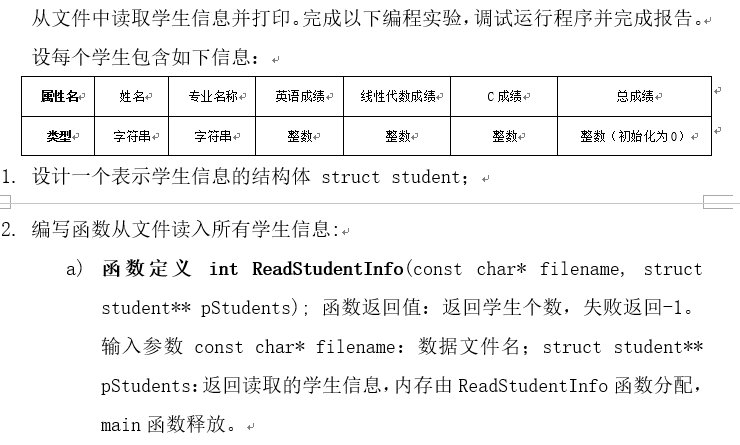b) 建议函数流程：
i. 打开数据文件，失败返回-1；
ii. 从头到尾扫描数据文件（即预读一遍）获得学生人数；
iii. 根据学生人数，利用malloc和sizeof等函数或运算符为struct student** pStudents分配内存；
iv. 利用rewind函数，定位到文件头；
v. 读取数据文件，并把每一条记录通过数组下标法或指针法保存到结构体数组pStudents中；
vi. 关闭文件；
vii. 返回学生人数。

c) 数据文件格式说明：

1. 编写统计函数：
a) 函数定义void Count(struct student pStudents[], int num)。输入参数分别为保存学生的结构体数组和学生人数。
b) 功能为：统计所有学生的总成绩和每个专业（假定一共三个专业：通信，网络，物联网）的平均成绩；输入参数包括学生信息结构体数组，学生人数，返回的每个学生的总成绩直接填充到对应的输入结构体数组对应字段中。统计信息包括三个专业的总人数、平均成绩数组，用全局变量来表示。访问每个学生信息的时候，用指针来指向当前正在处理的学生。
c) 设计一个或者三个带参数的宏，判断输入的字符串是否是指定的专业名称，并在函数Count中使用。

2. 编写main函数实现：
a) 定义变量，其中保存学生信息的数据文件直接在源代码中初始化；
b) 读取学生信息，如果失败，打印失败信息并退出程序；
c) 统计学生信息；
d) 输出统计信息。
e) 释放内存。

``````#include <stdio.h>
#include <stdlib.h>
#include <string.h>

struct student{
char name;
char major;
int Eng_sco;
int cal_sco;
int c_sco;
int sum;
};
int tx_per=0,tx_ave,tx_sum=0;
int wl_per=0,wl_ave,wl_sum=0;
int wlw_per=0,wlw_ave,wlw_sum=0;
int ReadStudentInfo(const char* filename, struct student** pStudents);
void Count(struct student pStudents[], int num);
int main()
{

int count;
int m;
struct student *list;
printf("学生信息： \n");
printf("----------------------------------------------------\n");
printf("序号  姓 名  专业名称 英语成绩 线性代数成绩 c语言成绩 总成绩\n");
for(m=1;m<=count;m++)
{
printf("%4d %s %s %4d %4d %4d %4d\n",m,(list+m-1)->name,(list+m-1)->major,(list+m-1)->Eng_sco,(list+m-1)->cal_sco,(list+m-1)->c_sco,(list+m-1)->sum);
}
Count(list, count);
printf("\n统计信息 ：\n");
printf("----------------------------------------------------\n");
printf("序号  专业名称 学生人数 平均成绩\n");
printf("   1    通信      %d       %d\n",tx_per,tx_ave);
printf("   2    网络      %d       %d\n",wl_per,wl_ave);
printf("   3   物联网     %d       %d\n",wlw_per,wlw_ave);

return 0;
}
int ReadStudentInfo(const char* filename, struct student** pStudents)
{
FILE *fp;
char ch;
int i=1,j;
if(fp==NULL)
{
printf("cann't open the file");
exit(-1);
}
ch=fgetc(fp);
while(ch!=EOF)
{
if(ch=='\n')
{
i++;
}
ch=fgetc(fp);
}
rewind(fp);
*pStudents=(struct student*)malloc(sizeof(** pStudents)*i);
for(j=0;j<i;j++)
{
(*pStudents+j)->sum=((*pStudents+j)->Eng_sco+(*pStudents+j)->cal_sco+(*pStudents+j)->c_sco);
}
fclose(fp);
return i;
}
void Count(struct student pStudents[], int num)
{
int n=1;
for(n=1;n<=num;n++)
{
if(!strcmp((pStudents+n-1)->major,"通信"))
{
tx_per++;
tx_sum=tx_sum+(pStudents+n-1)->sum;
}
if(!strcmp((pStudents+n-1)->major,"网络"))
{
wl_per++;
wl_sum=wl_sum+(pStudents+n-1)->sum;
}
if(!strcmp((pStudents+n-1)->major,"物联网"))
{
wlw_per++;
wlw_sum=wlw_sum+(pStudents+n-1)->sum;
}
tx_ave=tx_sum/tx_per;
wl_ave=wl_sum/wl_per;
wlw_ave=wlw_sum/wlw_per;
}
free(pStudents);
}

``````

13个回答

`````` \#include <stdio.h>
#include <stdlib.h>
#include <string.h>

struct student{
char name;
char major;
int Eng_sco;
int cal_sco;
int c_sco;
int sum;
};
int tx_per=0,tx_ave,tx_sum=0;
int wl_per=0,wl_ave,wl_sum=0;
int wlw_per=0,wlw_ave,wlw_sum=0;
int ReadStudentInfo(const char* filename, struct student** pStudents);
void Count(struct student pStudents[], int num);
int main()
{

int count;
int m;
struct student *list;
printf("学生信息： \n");
printf("----------------------------------------------------\n");
printf("序号  姓名  专业名称  英语成绩   线性代数成绩    c语言成绩   总成绩\n");
for(m=1;m<=count;m++)
{
printf("%4d %6s %6s %4d %4d %4d %4d\n",m,(list+m-1)->name,(list+m-1)->major,(list+m-1)->Eng_sco,(list+m-1)->cal_sco,(list+m-1)->c_sco,(list+m-1)->sum);
}
Count(list, count);
printf("\n统计信息 ：\n");
printf("----------------------------------------------------\n");
printf("序号  专业名称 学生人数 平均成绩\n");
printf("   1    通信      %d       %d\n",tx_per,tx_ave);
printf("   2    网络      %d       %d\n",wl_per,wl_ave);
printf("   3   物联网     %d       %d\n",wlw_per,wlw_ave);

return 0;
}
void CutString(char* buf,char*temp,char sp);
int ReadStudentInfo(const char* filename, struct student** pStudents)
{
FILE *fp;
char ch;
int i=1,j;
fp=fopen(filename,"r");
if(fp==NULL)
{
printf("cann't open the file");
exit(-1);
}
ch=fgetc(fp);
while(ch!=EOF)
{
if(ch=='\n')
{
i++;
}
ch=fgetc(fp);
}
rewind(fp);
*pStudents=(struct student*)malloc(sizeof(** pStudents)*i);

char buf={0};
for(j=0;j<i;j++)
{
memset(*pStudents+j,0,sizeof(struct student));
fgets(buf,512,fp);
int len = strlen(buf);
buf[len]=' ';

CutString(buf,(*pStudents+j)->name,' ');
CutString(buf,(*pStudents+j)->major,' ');
char e_Score,cal_Score,c_Score;

memset(e_Score,0,8);
memset(cal_Score,0,8);
memset(c_Score,0,8);
CutString(buf,e_Score,' ');
(*pStudents+j)->Eng_sco = atoi(e_Score);
CutString(buf,cal_Score,' ');
(*pStudents+j)->cal_sco = atoi(cal_Score);
CutString(buf,c_Score,' ');
(*pStudents+j)->c_sco = atoi(c_Score);
(*pStudents+j)->sum=((*pStudents+j)->Eng_sco+(*pStudents+j)->cal_sco+(*pStudents+j)->c_sco);
}
fclose(fp);
return i;
}

void CutString(char* buf,char*temp,char sp)
{
int len = strlen(buf);
for (int i=0;i<len;i++)
{
if (buf[i]==sp)
{
memcpy(buf,buf+i+1,strlen(buf+i+1));
break;
}
temp[i] = buf[i];
}
}

void Count(struct student pStudents[], int num)
{
int n=1;
for(n=1;n<=num;n++)
{
if(!strcmp((pStudents+n-1)->major,"通信"))
{
tx_per++;
tx_sum=tx_sum+(pStudents+n-1)->sum;
tx_ave=tx_sum/tx_per;
}
if(!strcmp((pStudents+n-1)->major,"网络"))
{
wl_per++;
wl_sum=wl_sum+(pStudents+n-1)->sum;
wl_ave=wl_sum/wl_per;
}
if(!strcmp((pStudents+n-1)->major,"物联网"))
{
wlw_per++;
wlw_sum=wlw_sum+(pStudents+n-1)->sum;
wlw_ave=wlw_sum/wlw_per;
}
}
free(pStudents);
}

``````senlijiu 我这样改，但就是运行不起来。我把fopen中的文件名改为一个不存在的文件名时，可以打印出cann't open the file然后跳出。但改为形参或存在的文件名时，程序就运行不起来

``````fp=fopen(readme.txt,"r");第一个参数路径需要双引号
``````

fp=fopen(filename,"r");c语言读取WAV文件并读取
c语言如何将WAV音频文件读取并存入char数组中，读入的数据要能用而不是乱码！求大神！

c# 如何从数据库格式（eg: .db , .sqlite）文件流中读取数据信息显示界面中，而不是直接从文件中读取
c# 如何从数据库格式（eg: .db , .sqlite）文件流中读取数据信息显示界面中，而不是直接从文件中读取，麻烦各位告知一下这种情况如何处理，谢谢

c语言如何读取文本文件中指定的字符串并保存到字符串数组中？

c语言如何删除文本文件的内容，和读取指定文件夹里的文件？

C语言大量文件依次读写

C语言读取excel或txt文件数据
C语言如何读取Excel或txt文件中有float型和字符串型数据中的float型数据
C语言文件读写读取不了
c语言 输入20个学生成绩

C语言程序 学生成绩排序系统
1. 学生成绩排序系统简介 学生成绩排序系统可实现学生信息输入、学生信息输出、学生信息查询、学生成绩排序等功能。程序要求提供简单友好易于操作的界面。程序以C语言开发。 2.学生成绩管理系统基本要求： 假设有5名学生的成绩放在1个数组中。系统要求从键盘上输入的方法生成学生的成绩几有关数据。系统能按照要求根据不同条件对学生成绩进行管理。 3. 系统模块及操作要求： （1） 编写一个成绩生成函数。使用从键盘上输入的方法生成学生的各门课程的成绩（每门课程的成绩都是0-100之间的整数）并存入文件中，通过调用该函数生成全部学生的成绩再从文件中读出数据。 （2）先采用冒泡排序法，将学生成绩从高到低进行排序，再输入一个学生的成绩，将此成绩按照排 序规律插入已排好序的学生成绩数组。 （3）在函数中进行 全班学生成绩从高到低的顺序进行排名, 排名方式根据上方一排好的顺序。 （4）对学生的成绩进行优化。 若遇到成绩相同的同学，按照学号的大小进行重新排序。 （5）定义一个结构体数组，存放学生的学号，姓名，三 门课的成绩，输出单门课成绩最高的学生的学号、姓名，输出三门课程的平均分数以及最高的学生的学号、姓名及其平均分，再将学生按照平均分数从高到低进行排序并输出最后的结果。 （6）学生成绩文件管理。将学生的相关数据，存入文件中，再从文件中读出 按照平均分数从高到低进行排序，将结果输出到屏幕上，再从文件中读取学生的数据进行显示。 设计一个菜单，至少具有上述操作要求的基本功能，菜单设计请见图1。![图片说明](https://img-ask.csdn.net/upload/201605/31/1464710124_909761.png)
c语言中文件的读取问题。
#include<stdio.h> #include<string.h> #include<stdlib.h> #define N 1 int main() { struct call { char name; char telephone; char type; }; struct call s1[N]; int i; FILE *fp; char filename; fp=fopen(filename,"w"); printf("请输入打开路径:\n"); gets(filename); if(fp==NULL) { printf("此路径打不开文件\n"); exit (0); } for(i=0;i<=N;i++) { printf("请输入第%d个人的信息\n",i+1); printf("请输入联系人的姓名:\n"); gets(s1[i].name); printf("请输入联系人的电话:\n"); gets(s1[i].telephone); printf("请输入联系人的类别:\n"); gets(s1[i].type); fwrite(&s1[i],sizeof(struct call),1,fp); } fclose(fp); fp=fopen(filename,"rt"); printf("请输入打开路径:\n"); gets(filename); printf("通讯录的数据为:\n"); printf("姓名 电话号码 类别\n"); i=0; while(fread(&s1[i],sizeof(struct call),1,fp)!=NULL) { printf("%s %s %s\n",s1[i].name,s1[i].telephone,s1[i].type); i++; } fclose(fp); return 0; } 将数据写去文件时，终端可以显示，但顺着路径找，文件里面没有，刚开始学习文件，所以有点不知所措，望指导指导！
C语言 文件读写fscanf 不能读取文件第一行

c语言读取文件出现一串乱码
C语言从学生档案文件中读取平时分并按某种计算出平时成绩并创建一个新文件输出

c语言实现txt文件读取
VC编译器下c语言没有办法打开TXT文件。代码如下：#include <stdio.h> #include<stdlib.h> void main() { FILE *fp; if((fp=fopen("C:\Users\Administrator\Desktop\ad.txt","r"))==NULL) { printf("cannot open the file!"); exit(0); } else printf("ok"); fclose(fp); }
Java语言如何读取txt学生成绩记录？txt文件放在作业05目录下，可能有好几个，读取哪一个
Java语言如何读取txt学生成绩记录？txt文件放在作业05目录下，可能有好几个，读取哪一个

《奇巧淫技》系列-python！！每天早上八点自动发送天气预报邮件到QQ邮箱

11月8日，由中国信息通信研究院、中国通信标准化协会、中国互联网协会、可信区块链推进计划联合主办，科技行者协办的2019可信区块链峰会将在北京悠唐皇冠假日酒店开幕。 　　区块链技术被认为是继蒸汽机、电力、互联网之后，下一代颠覆性的核心技术。如果说蒸汽机释放了人类的生产力，电力解决了人类基本的生活需求，互联网彻底改变了信息传递的方式，区块链作为构造信任的技术有重要的价值。 　　1...

C语言魔塔游戏

Android性能优化(4)：UI渲染机制以及优化

Python爬虫爬取淘宝，京东商品信息

Java工作4年来应聘要16K最后没要,细节如下。。。

SpringBoot2.x系列教程（三十六）SpringBoot之Tomcat配置
Spring Boot默认内嵌的Tomcat为Servlet容器，关于Tomcat的所有属性都在ServerProperties配置类中。同时，也可以实现一些接口来自定义内嵌Servlet容器和内嵌Tomcat等的配置。 关于此配置，网络上有大量的资料，但都是基于SpringBoot1.5.x版本，并不适合当前最新版本。本文将带大家了解一下最新版本的使用。 ServerProperties的部分源...
Python绘图，圣诞树，花，爱心 | Turtle篇

CPU对每个程序员来说，是个既熟悉又陌生的东西？ 如果你只知道CPU是中央处理器的话，那可能对你并没有什么用，那么作为程序员的我们，必须要搞懂的就是CPU这家伙是如何运行的，尤其要搞懂它里面的寄存器是怎么一回事，因为这将让你从底层明白程序的运行机制。 随我一起，来好好认识下CPU这货吧 把CPU掰开来看 对于CPU来说，我们首先就要搞明白它是怎么回事，也就是它的内部构造，当然，CPU那么牛的一个东...

2020年全新Java学习路线图，含配套视频，学完即为中级Java程序员！！

B 站上有哪些很好的学习资源?

Web播放器解决了在手机浏览器和PC浏览器上播放音视频数据的问题，让视音频内容可以不依赖用户安装App，就能进行播放以及在社交平台进行传播。在视频业务大数据平台中，播放数据的统计分析非常重要，所以Web播放器在使用过程中，需要对其内部的数据进行收集并上报至服务端，此时，就需要对发生在其内部的一些播放行为进行事件监听。 那么Web播放器事件监听是怎么实现的呢？ 01 监听事件明细表 名...
3万字总结，Mysql优化之精髓

HTML5适合的情人节礼物有纪念日期功能